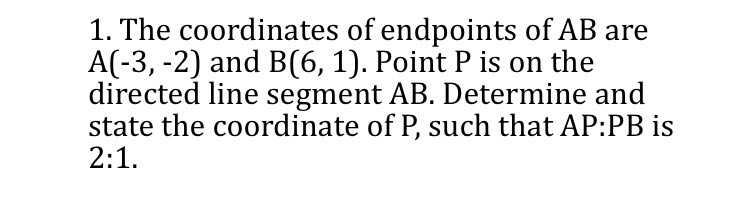### ¿Todavía tienes preguntas de matemáticas?

Pregunte a nuestros tutores expertos
Algebra
Pregunta1. The coordinates of endpoints of $$A B$$ are $$A ( - 3 , - 2 )$$ and $$B ( 6,1 )$$ . Point $$P$$ is on the directed line segment $$A B$$ . Determine and state the coordinate of $$P$$ , such that AP:PB is $$2 : 1 .$$